# Texas Go Math Kindergarten Lesson 15.2 Answer Key Nickel

Refer to our Texas Go Math Kindergarten Answer Key Pdf to score good marks in the exams. Test yourself by practicing the problems from Texas Go Math Kindergarten Lesson 15.2 Answer Key Nickel.

## Texas Go Math Kindergarten Lesson 15.2 Answer Key Nickel

Essential Question
How do you identify, describe, and name nickels?

Explore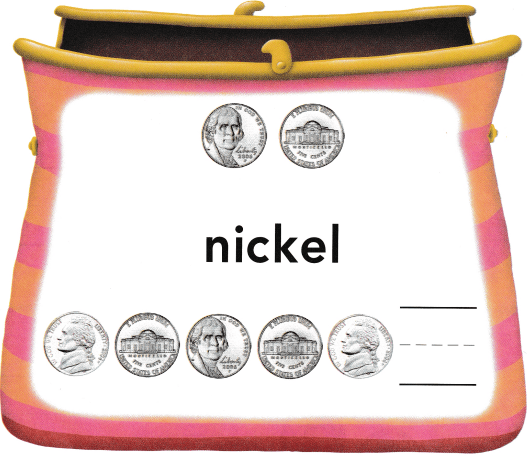Explanation:
A nickel is a five-cent coin struck by the United States
Nickel = 5 cents

Directions
Place a nickel to match each one shown at the top of the page. Tell what is alike about the nickels. Tell what is different about the nickels. Count the set of nickels at the bottom of the page. Write the number of nickels.

Share and Show

Question 1.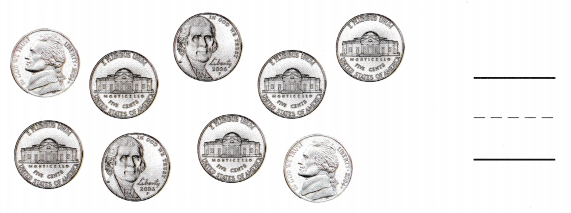Explanation:
1 nickel = 5 cents
There are 9 nickels

Question 2.Explanation:
1 nickel = 5 cents
There are 11 nickels

Question 3.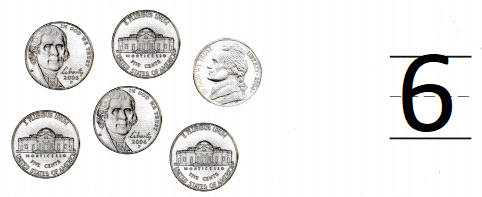Explanation:
1 nickel = 5 cents
There are 6 nickels

Directions
1-3. Count the nickels. Write the number that shows how many nickels.

Question 4.Explanation:
1 nickel = 5 cents
there are 8 nickels

Question 5.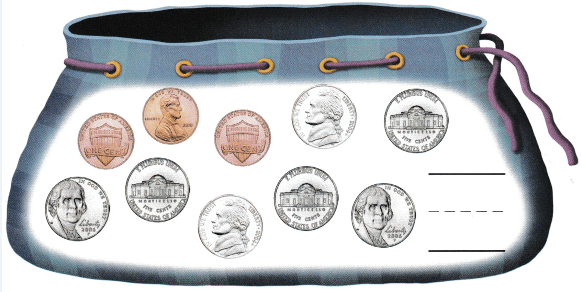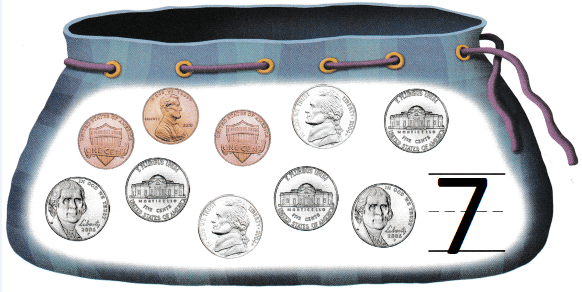Explanation:
1 nickel = 5 cents
There are 7 nickels

Directions
4-5. Circle the nickels. Count and write the number of nickels.

Home Activity

• Show your child a nickel. Hove him or her tell you the name of the coin and describe both sides.

Problem Solving

Question 6.Explanation:
Grayson needs 5 nickels to buy a snack.
Yes the money bag has 5 nickels
so, Grayson can buy the snack.

Question 7.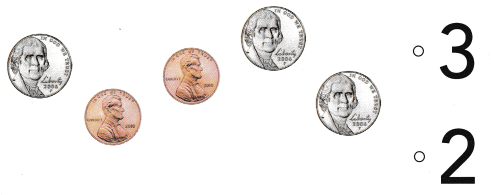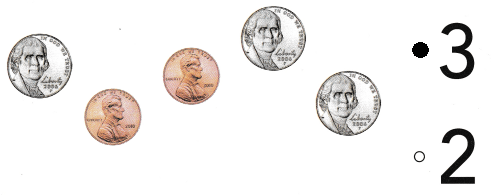Explanation:
1 nickel = 5 cents
There are 3 nickels

Directions
6. Grayson needs 5 nickels to buy a snack. He counts the nickels to see if he has enough. Circle the nickels, Write the number of nickels. 7. Choose the correct answer. How many nickels are in this group?

### Texas Go Math Kindergarten Lesson 15.2 Homework and Practice

Question 1.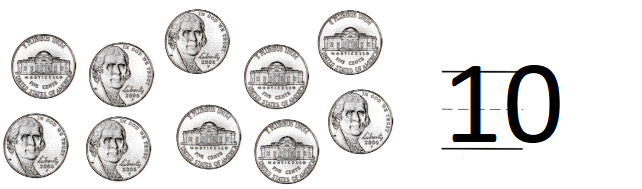Explanation:
1 nickel = 5 cents
There are 10 nickels.

Question 2.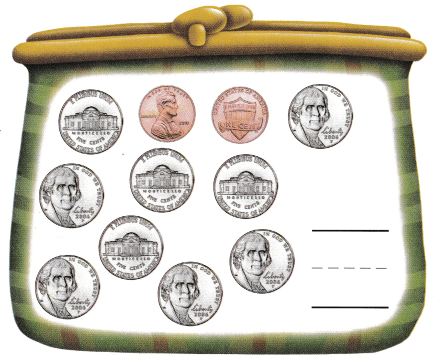Explanation:
The money bag has 9 nickels
and 2 pennies
1 nickel = 5 cents

Directions
1. Count the nickels. Write the number that shows how many nickels. 2. Circle the nickels. Count and write the number of nickels.

Texas test Prep

Lesson Check

Question 3.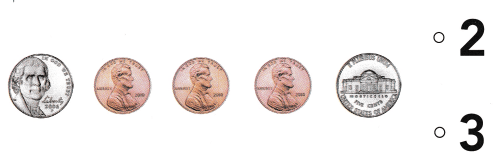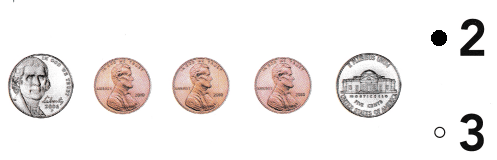Explanation:
There are 3 pennies
There are 2 nickels

Question 4.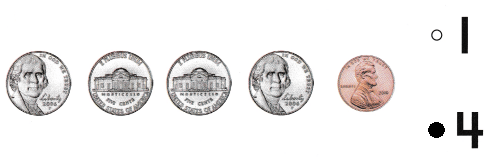Explanation:
There is 1 penny
There are 4 nickels

Question 5.